# 单表查询

## 选择表中的若干列

### 查询指定列

``````查询全体学生的学号和姓名
``````
``````Select SNAME,SNO FROM STUDENT
``````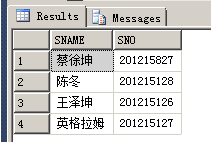### 查询全部列

``````Select * FROM STUDENT
``````

``````Select Sno,Sname,ssex,sage,sdept FROM STUDENT
``````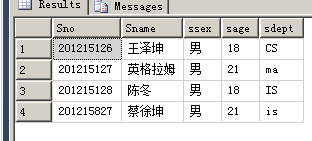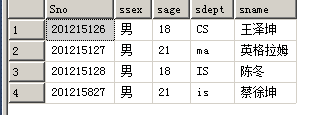### 查询经过计算的值

``````查询全体学生的姓名以及其出生年份
Select子句的目标表达式不仅可以是表中的属性列，也可以是表达式。
``````
``````Select Sname,2021-SAGE FROM STUDENT
````````````Select Sname,'出生年份',2021-sage,LOWER(Sdept) FROM STUDENT
``````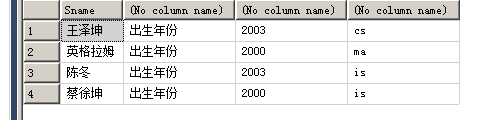``````Select Sname '姓名','出生年份'Birth,2021-sage 年龄,LOWER(Sdept) 专业 FROM STUDENT
``````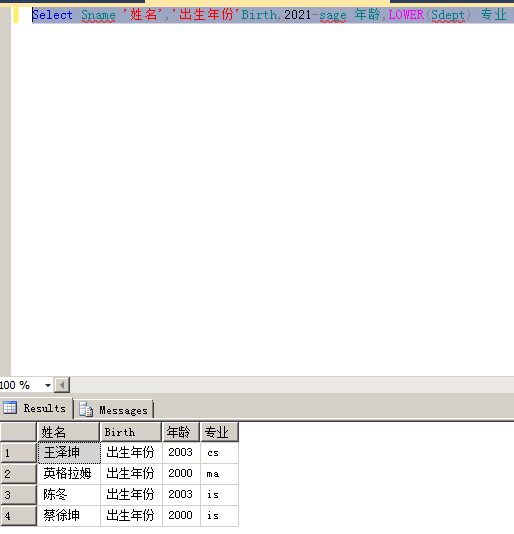## 选择表中的若干元组

### 消除取值重复的行

``````Select 2021-sage  FROM STUDENT
``````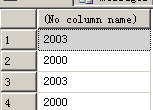``````Select DISTINCT 2021-sage  FROM STUDENT
``````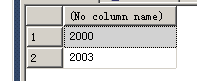``````Select 2021-sage  FROM STUDENT
``````

``````Select ALL 2021-sage  FROM STUDENT
``````

### 查询满足条件的元组

``````通过ｗｈｅｒｅ子句来实现
１》　比较大小
进行比较的运算符一般包括　＝　＞　＜　＞＝　＜＝　！＝　＜＞（不等于）　！＞　！＜
查询ｉｓ系全体学生的名单

``````
``````Select Sname from Student where Sdept ='is';
``````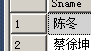``````Select Sname,Sage from Student where sAGE<20;
``````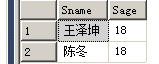2>> 确定范围

``````查询年龄在18-20岁之间的学生的姓名 专业和年龄
``````
``````Select Sname,SDEPT,SAGE from Student where Sage Between 18 And 20;
``````

3》 确定集合

``````Select Sname,Ssex from Student where Sdept IN ('CS','ma','is');
``````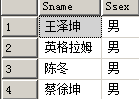4》 字符匹配

[NOT] LIKE ‘<匹配串>’ [ESCAPE ‘<转码字符>’]

• _ 代表任意单个字符 例如a_b代表以a开头 以b结尾的长度为3的任意字符串 比如acb adb

``````Select Sname,Ssex ,Sno from Student where Sname like '%坤';
``````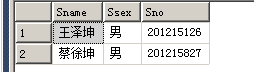``````Select Sname from Student where Sname like '蔡__';
``````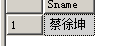``````Select Sname,sno,ssex from Student where Sname NOT like '蔡%';
``````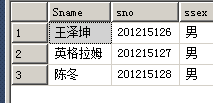``````select cno from Course where cname like 'DB\_Design' Escape '\';
``````

``````select Sno,Sname from Student where Sname like '王\_%' Escape '\';
``````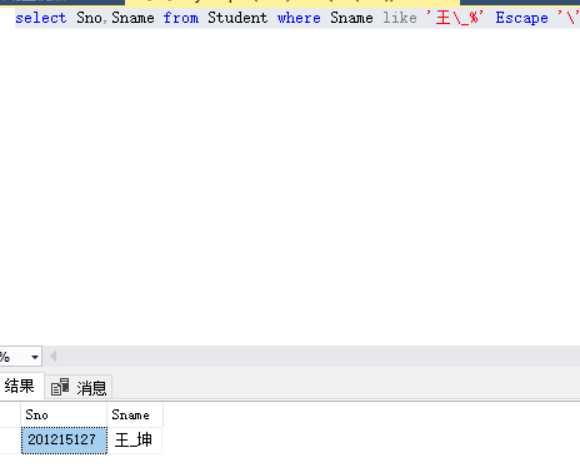5》 涉及空值的查询

``````select * from Student Where Sage is NULL;
``````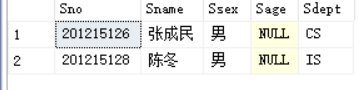``````select * from Student Where Sage is NOT NULL;
``````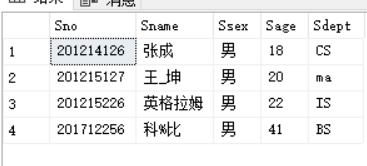6》多重条件查询

``````select Sname from Student Where Sdept='cs' And sage < 20;
``````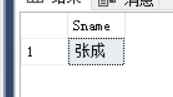``````Select Sname,Ssex from Student where Sdept IN ('CS','ma','is');
``````

``````Select Sname,Ssex from Student where Sdept ='CS'or Sdept ='ma'or Sdept ='is';
``````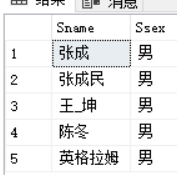## ORDER BY

``````Select Sname,Sdept from Student where Ssex='男'
Order by Sage DESC;
``````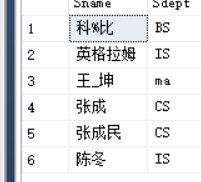``````Select * from Student
Order by Sdept,Sage DESC;
``````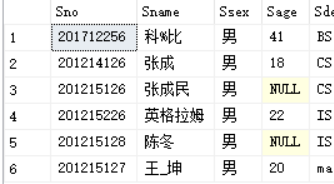## 聚集函数：

• 统计元组个数 COUNT(*) 统计一列中值的个数
• COUNT([DISTINCT|ALL] <列名>) 计算一列值的总和SUM([DISTINCT|ALL] <列名>) 计算一列值的平均值 AVG([DISTINCT|ALL] <列名>) 求一列中的最大值和最小值 MAX([DISTINCT|ALL] <列名>) MIN([DISTINCT|ALL] <列名>)
查询学生总人数：
``````Select Count(*) From Student;
``````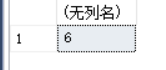``````Select AVG(SAGE) From Student where Sdept='cs';
``````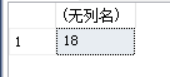``````Select MAX(SAGE) From Student where Sdept='IS';
``````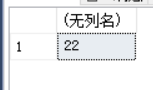``````Select SUM(SAGE) From Student where Sdept='CS';
``````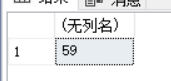## GROUP BY 子句

GROUP BY 子句查询结果按某一列或者多列的值分组，值相等的为一组

``````Select CNO,COUNT(SNO)
FROM SC
GROUP BY CNO;
``````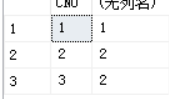``````Select CNO,COUNT(SNO)
FROM SC
GROUP BY CNO
HAVING COUNT(*) >=2;
``````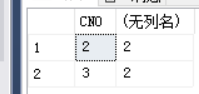``````Select CNO,AVG(GRADE)
FROM SC
GROUP BY CNO
``````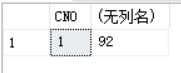Where 来进行筛选

Where 作用于基本表或视图，从中筛选满足元素的组，